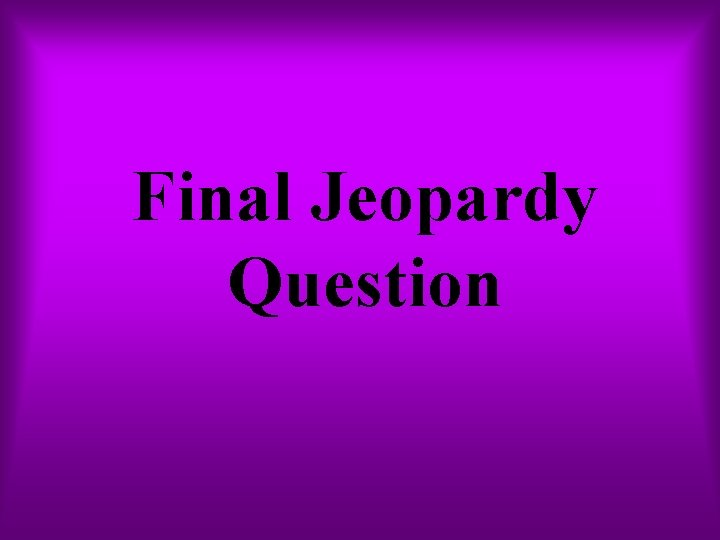# Probability Jeopardy Spinners Dice Marbles Coins Average 1

• Slides: 54Probability JeopardySpinners Dice Marbles Coins Average \$1 \$1 \$1 \$2 \$2 \$2 \$5 \$5 \$5 \$10 \$10 \$10 \$20 \$20 \$20 Probability Terms SituationsWhat is the probability of landing on a number under five? 1 2 4 3 Certain, possible, or impossible?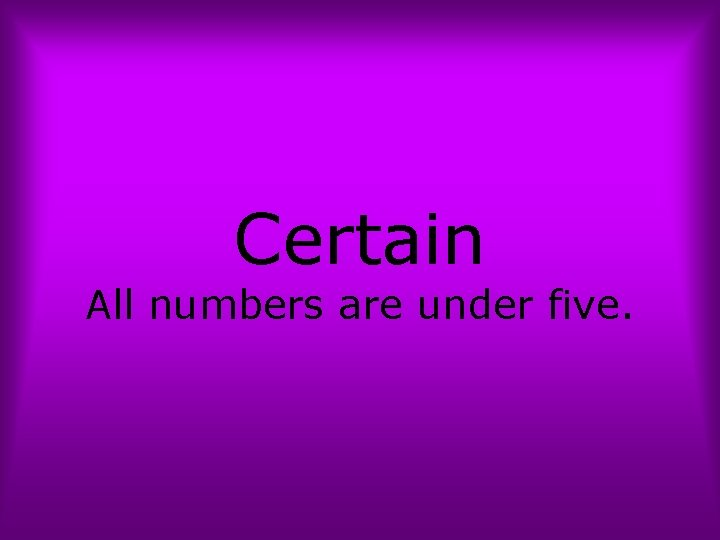Certain All numbers are under five.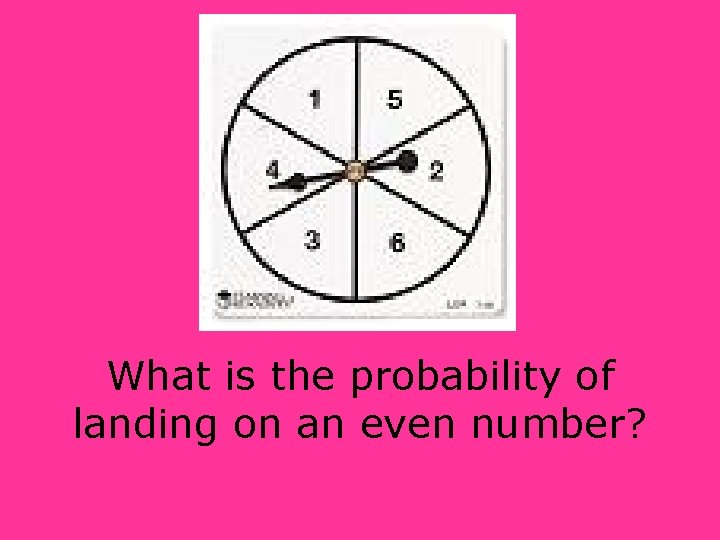What is the probability of landing on an even number?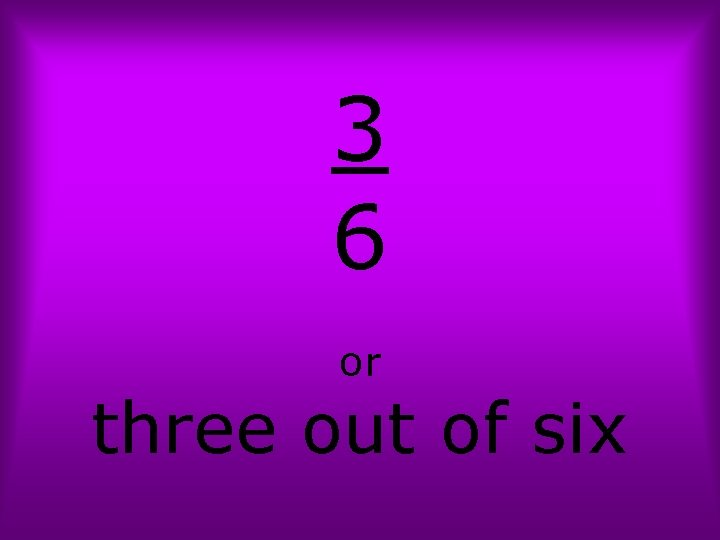3 6 or three out of six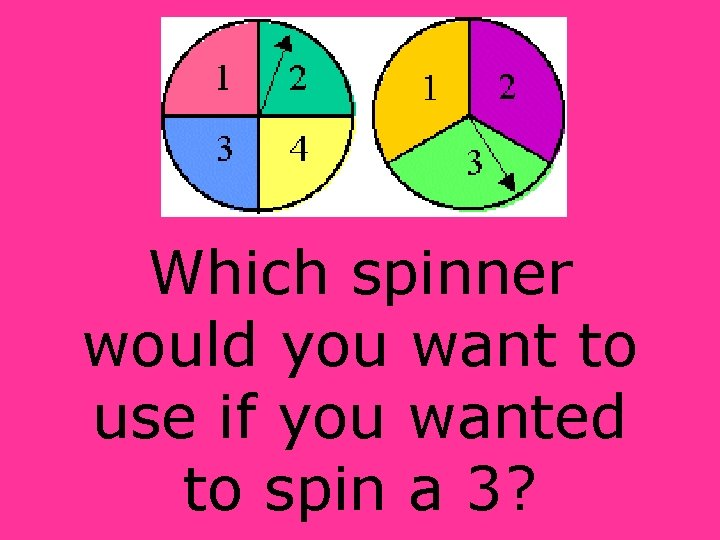Which spinner would you want to use if you wanted to spin a 3?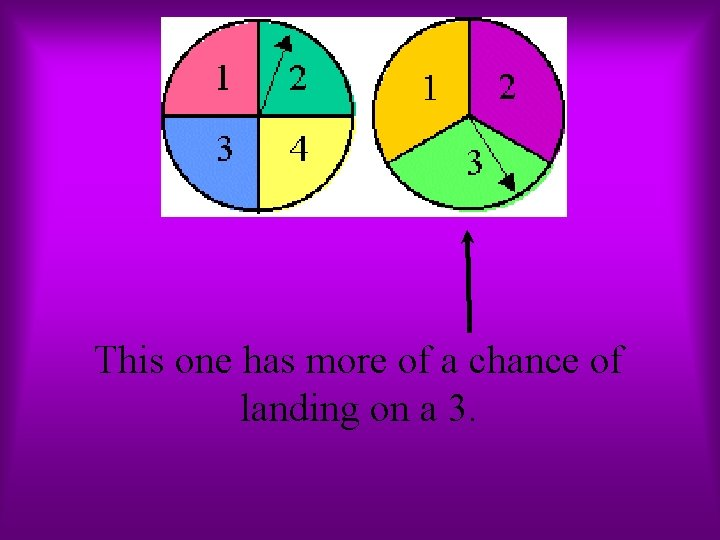This one has more of a chance of landing on a 3.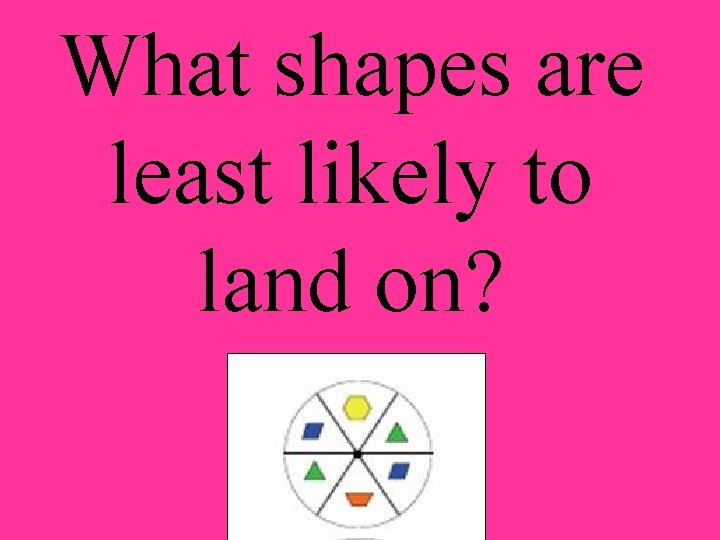What shapes are least likely to land on?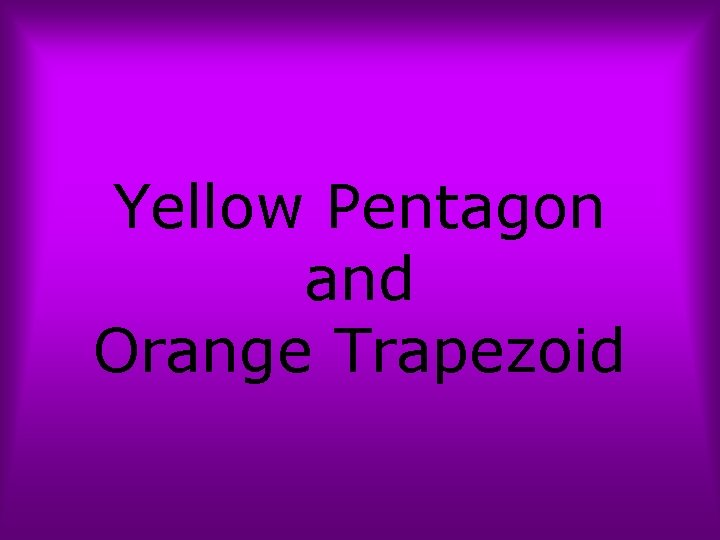Yellow Pentagon and Orange TrapezoidWhich spinner would you use if you wanted it to be equally like to spin white and blue?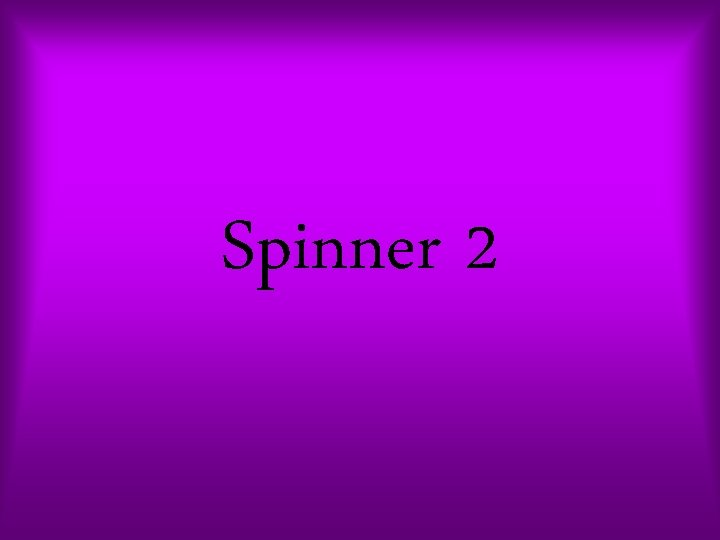Spinner 2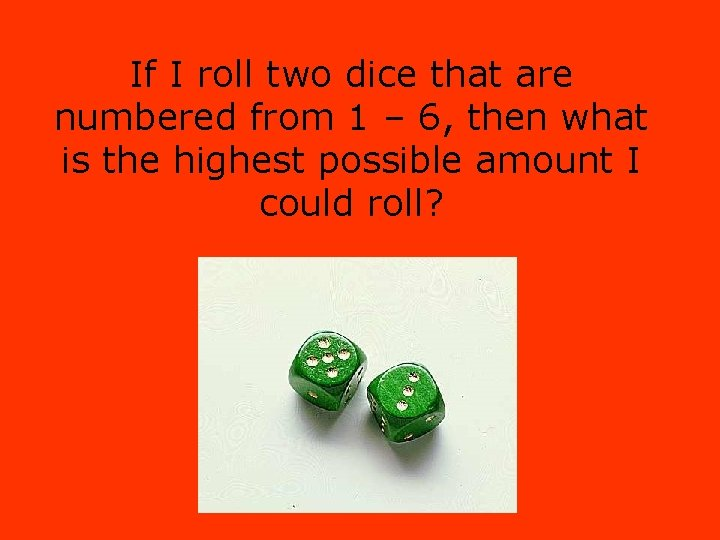If I roll two dice that are numbered from 1 – 6, then what is the highest possible amount I could roll?12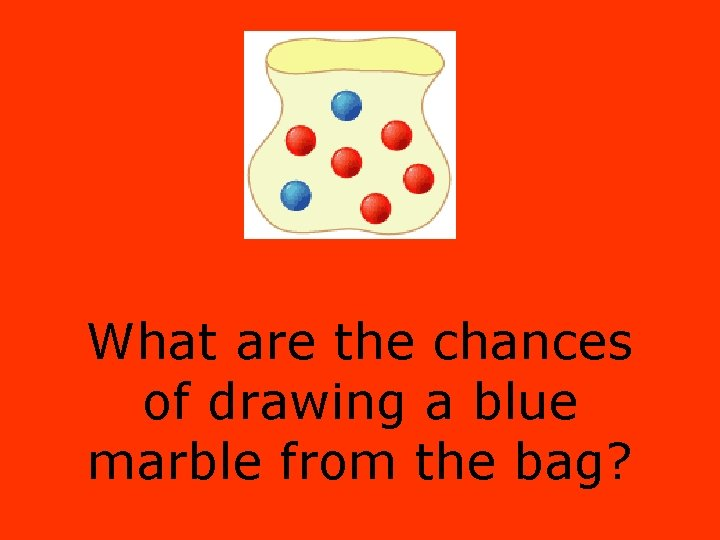What are the chances of drawing a blue marble from the bag?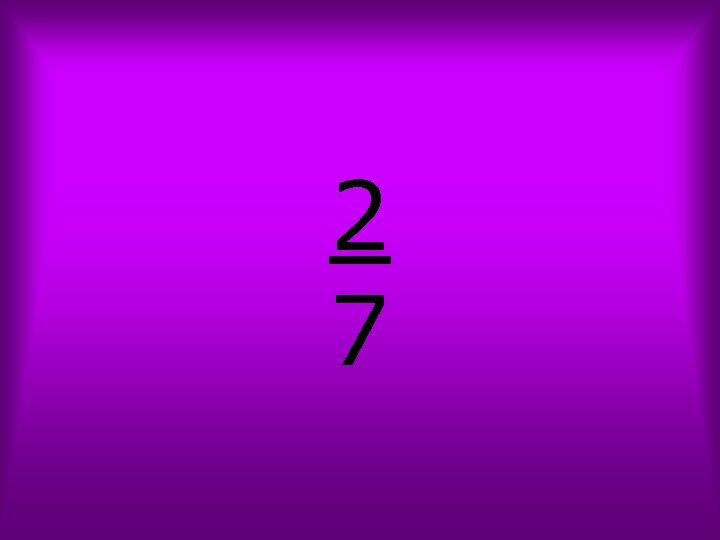2 7What type of marble has a three out of ten chance of being drawn from the bag?White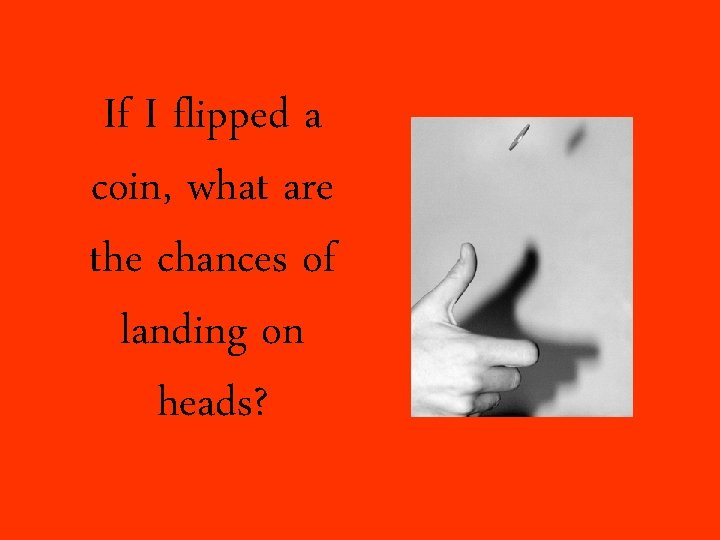If I flipped a coin, what are the chances of landing on heads?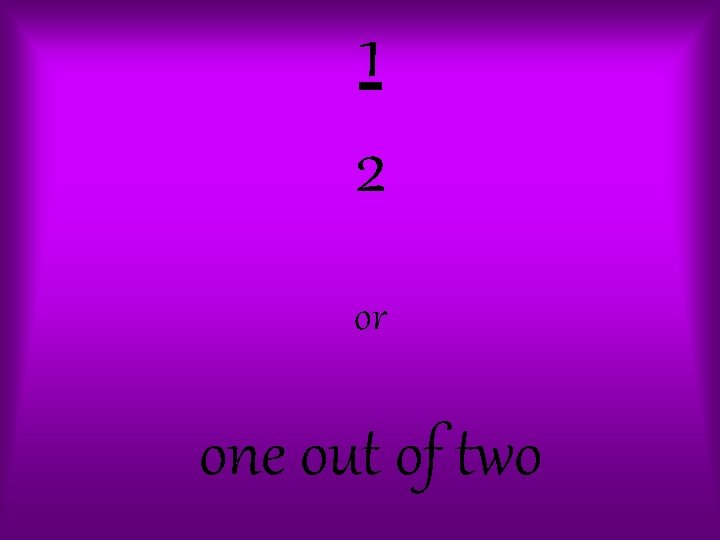1 2 or one out of twoI am rolling two dice and adding their totals together. One dice lands on a four. Is it certain, possible, or impossible to roll an amount higher than nine?Possible: If I roll a six with the second dice, then I would have a total higher than nine.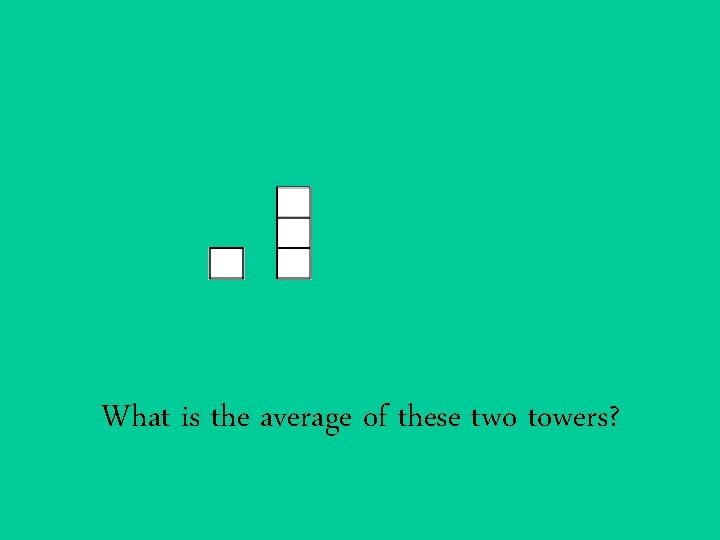What is the average of these two towers?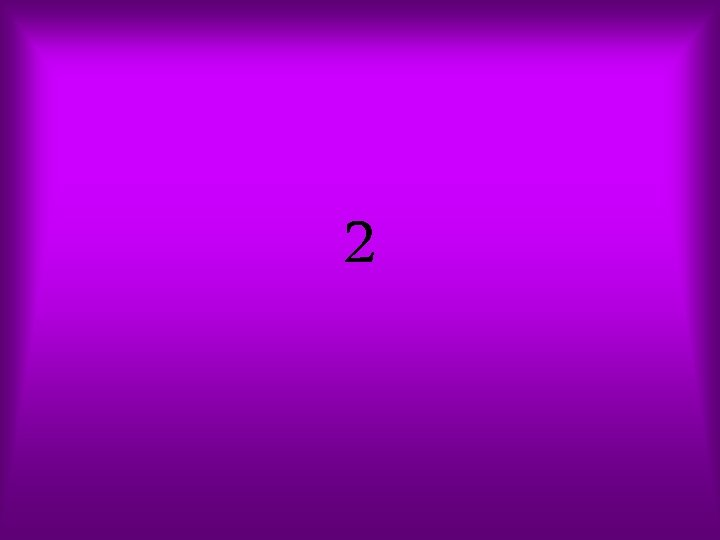2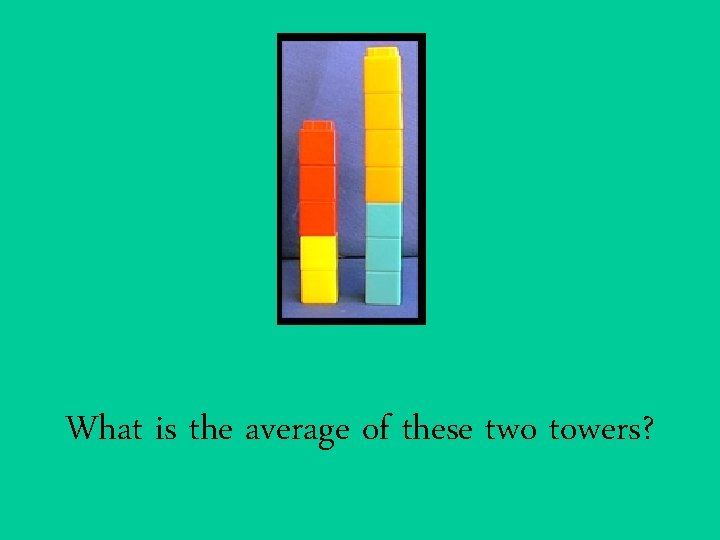What is the average of these two towers?6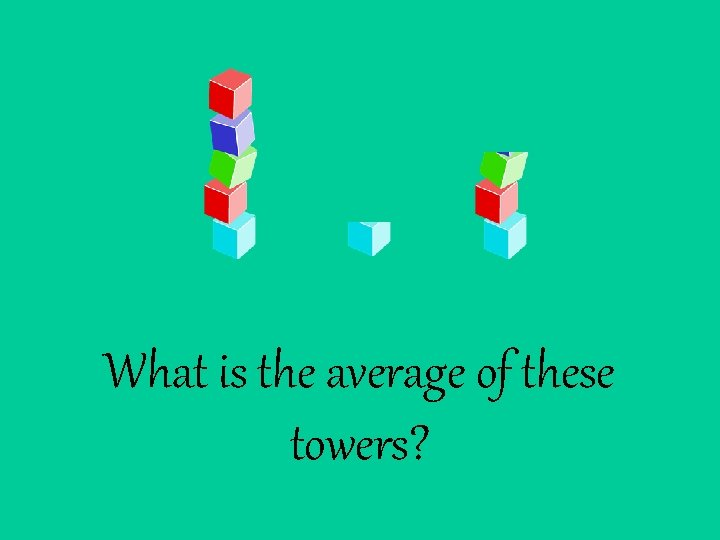What is the average of these towers?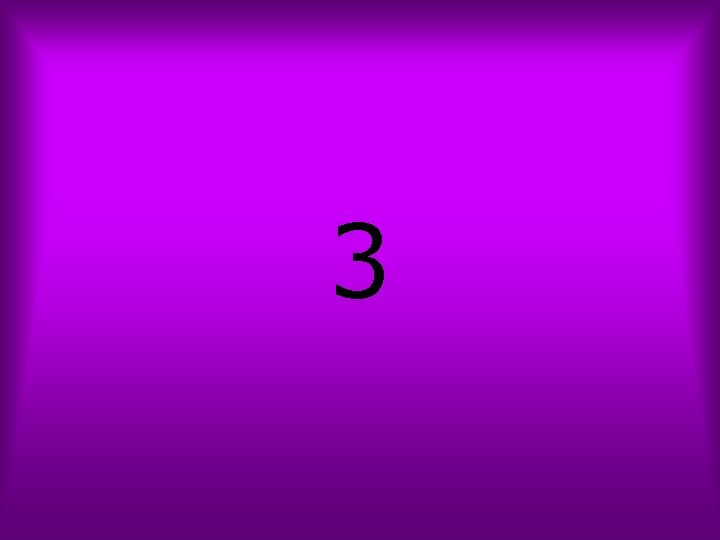3How would you write a math problem to find the average of these three towers?5+1+3=9 9÷ 3=3 the average is three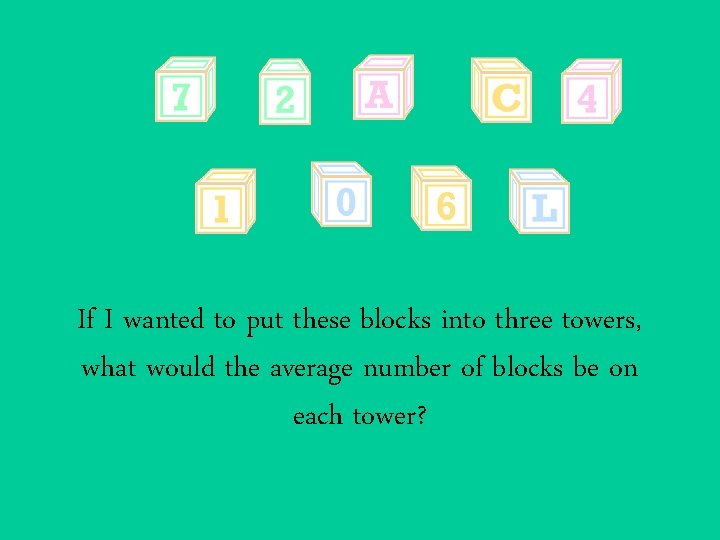If I wanted to put these blocks into three towers, what would the average number of blocks be on each tower?The average would be three.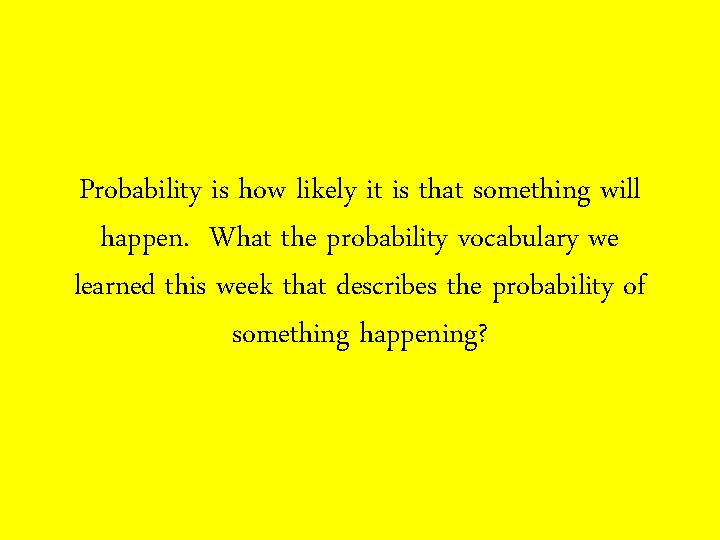Probability is how likely it is that something will happen. What the probability vocabulary we learned this week that describes the probability of something happening?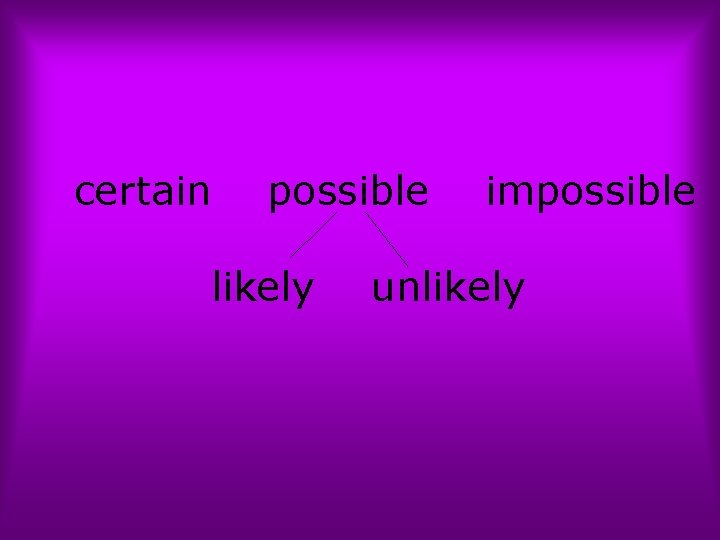certain possible likely impossible unlikely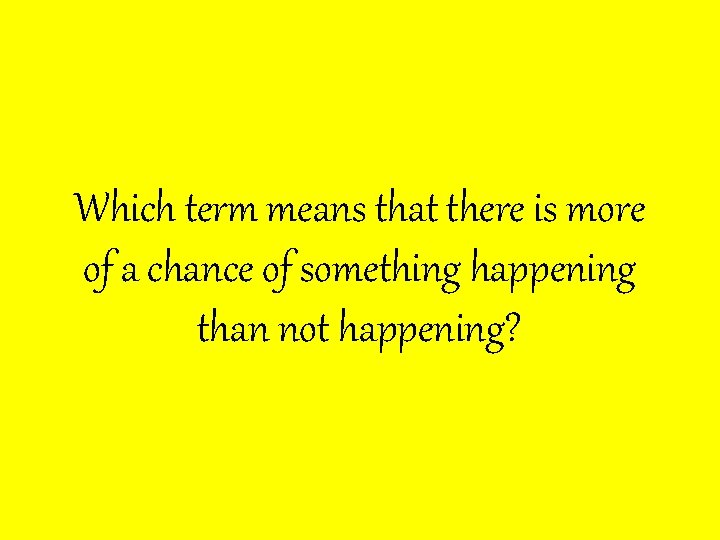Which term means that there is more of a chance of something happening than not happening?Likely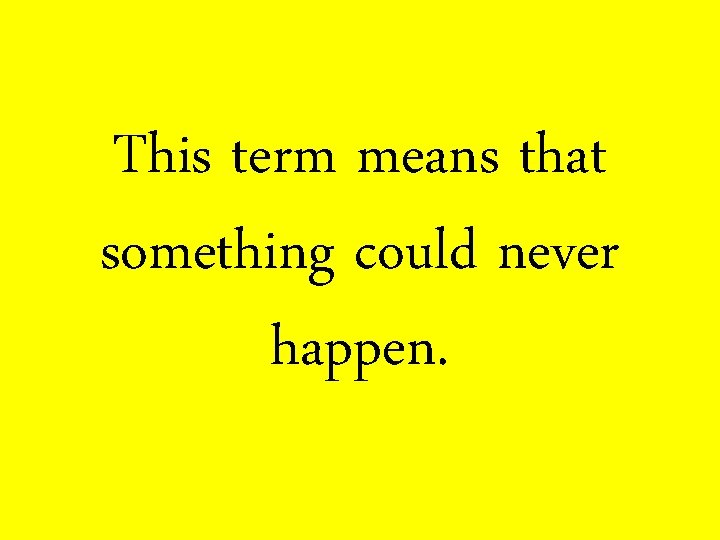This term means that something could never happen.impossible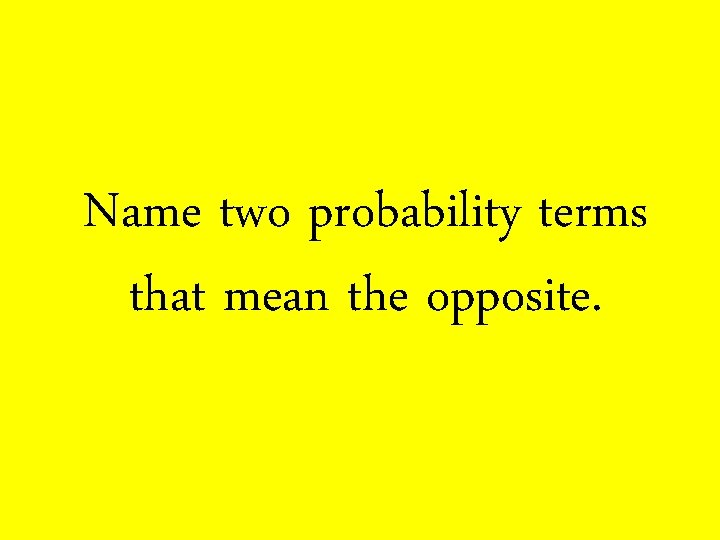Name two probability terms that mean the opposite.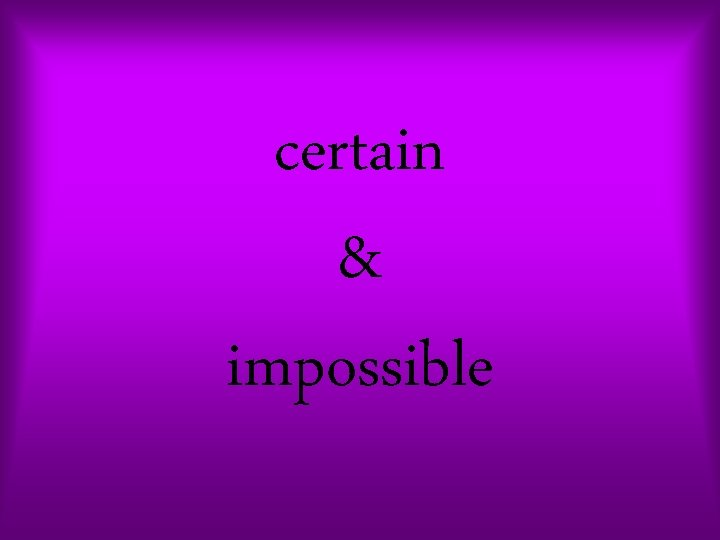certain & impossible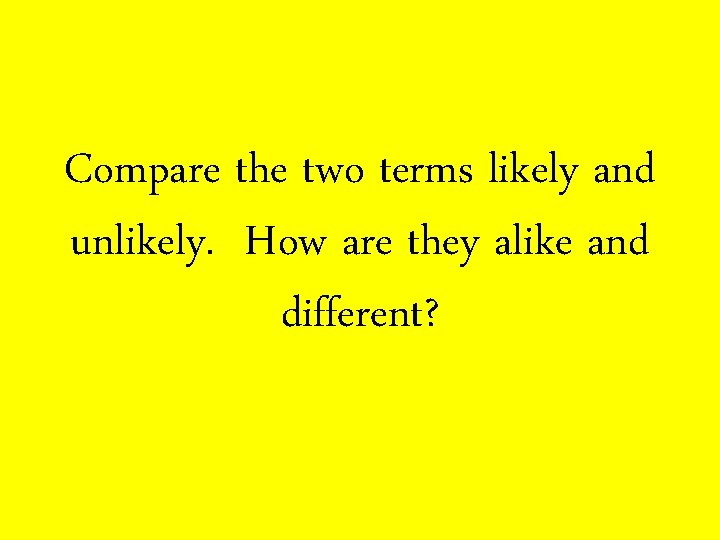Compare the two terms likely and unlikely. How are they alike and different?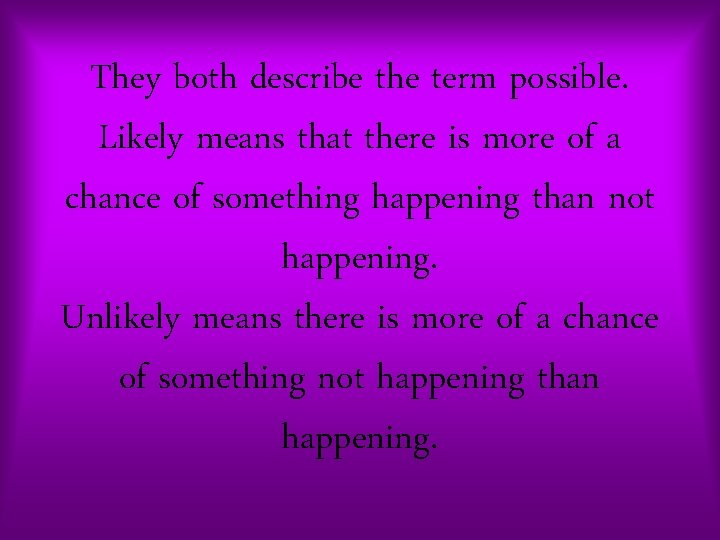They both describe the term possible. Likely means that there is more of a chance of something happening than not happening. Unlikely means there is more of a chance of something not happening than happening.What are the chances that you can skip fourth grade next year and go to fifth instead?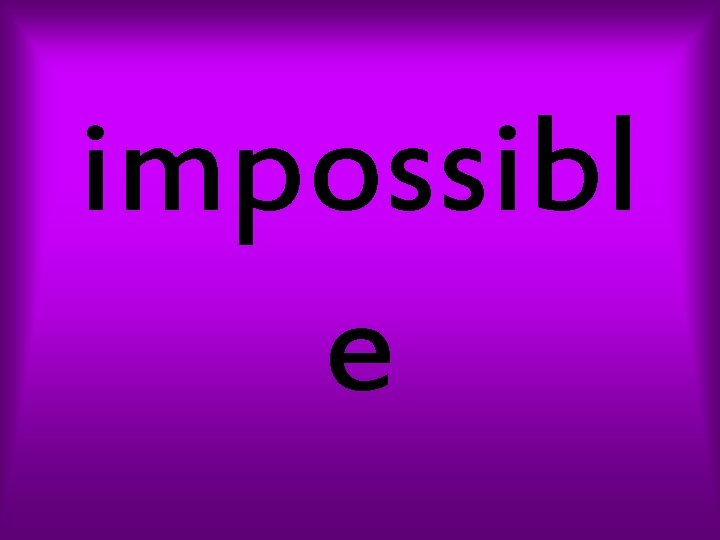impossibl e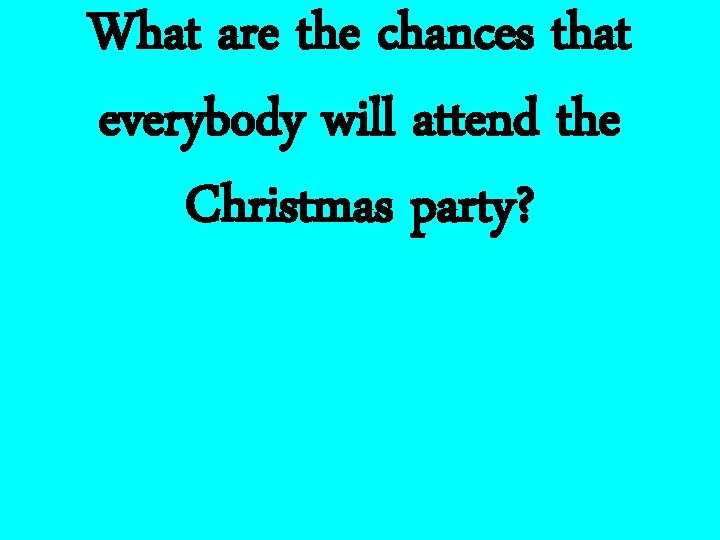What are the chances that everybody will attend the Christmas party?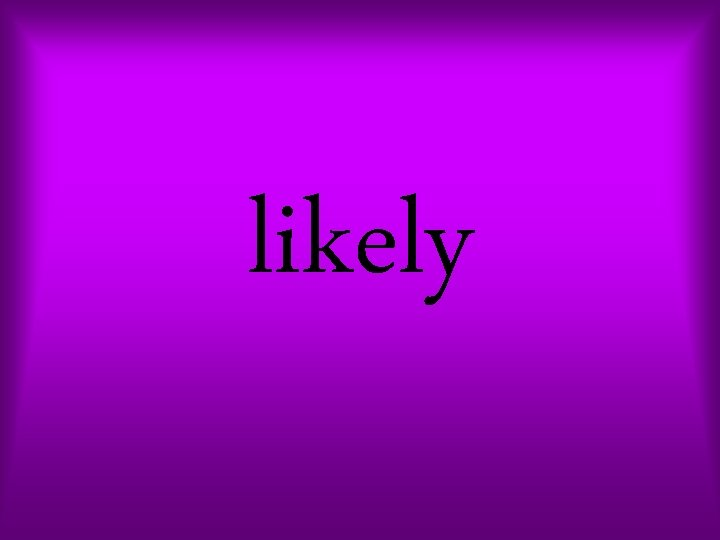likely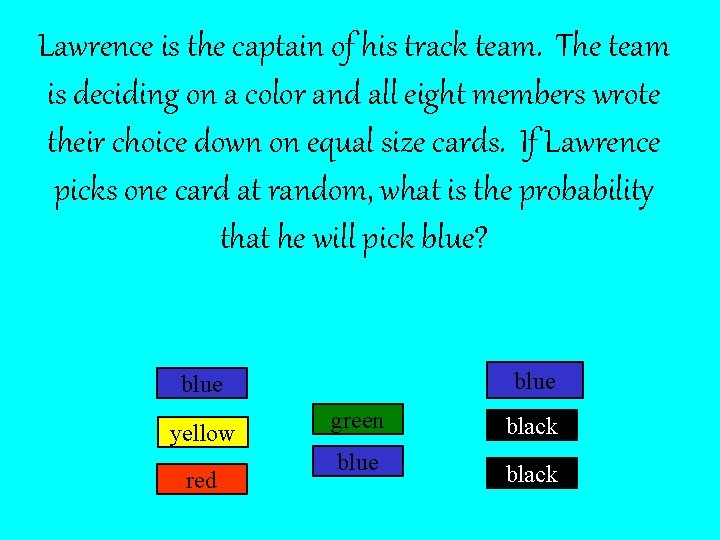Lawrence is the captain of his track team. The team is deciding on a color and all eight members wrote their choice down on equal size cards. If Lawrence picks one card at random, what is the probability that he will pick blue? blue yellow red green black blue black3 out of 8 chance or 3 8If you have to land on an even number to get a point. Then how many different results would give you a point?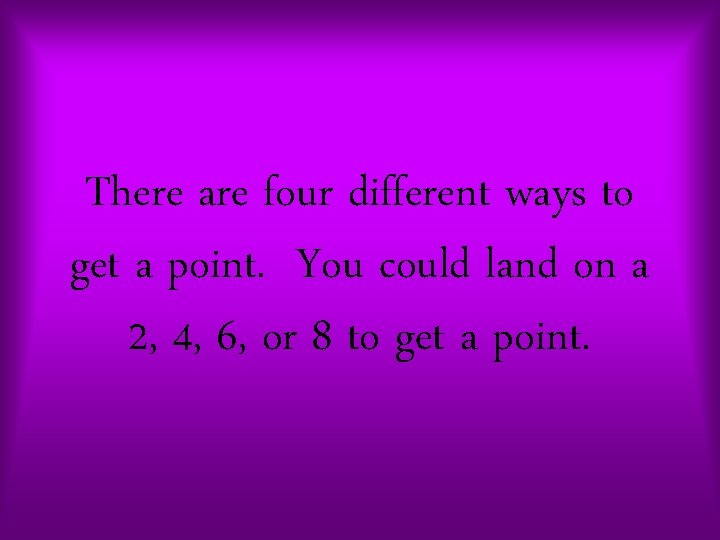There are four different ways to get a point. You could land on a 2, 4, 6, or 8 to get a point.When a meteorologist states that the chance of rain is 50%, the meteorologist is saying that it is equally likely to rain or not to rain. If the chance of rain rises to 80%, what is the probability of it raining?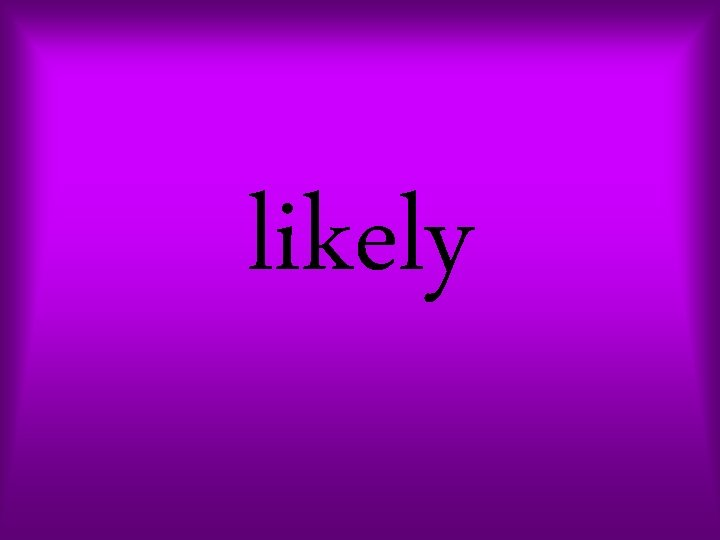likely